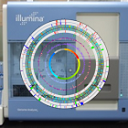#Genome Informatics Laboratory at KRIBB

### Sidebar

This is the sidebar. Without it, the main text is too wide!

2019년 11월 교육 자료

2.rnaseq
```##### installing packages example (will not do in workshop)
tr()
setRepositories()
install.packages("psych")
library(psych)
tr()
m <- matrix(1:16,ncol=4)
tr(m)
#####################################

#################
#  Basic plots  #
#################
x11(width = 6, height = 6)   #code to open up a window in linux
counts <- matrix( rnorm(200,mean=0,sd=1),20,10 )   #make a normal count
boxplot( counts[,1],counts[,3],counts[,5],counts[,7],counts[,9],counts[,10] , col=c("black","blue","green","red","purple","yellow") )
boxplot( counts[,1],counts[,3],counts[,5],counts[,7],counts[,9],counts[,10] , col=c("red","red","red","blue","blue","blue") )
test_data <- c(1,2,2,3,7,1,2,3,10,11,25,34,3,6,7,9,9,10,10,10)  #make test data
hist(test_data)
test_data <- rnorm(500, mean = 0, sd = 1)
hist(test_data)
hist(test_data, main = "Histogram of Test Dataset", xlab = "Trait", ylab = "Individual Counts" )
dev.off() #or close the window

#실습 1교시 마무리.

## Set the working directory (not needed for workshop)
#setwd("C:\\PATH\\") ## in pc
#setwd("/home/2.RNASeq_practice/5.HTseq_simulated")   ##in linux

## Call necessary libraries (these are preinstalled [ex:install.packages("limma")])
library(statmod)
library(locfit)
library(edgeR)
library(limma)
library(DESeq2)

## note the bad samples (6 and 10)
## save used samples' index to Sam_Index
Samp_Index <- c(1:5,7:9,11:23)

## or you can do
Samp_Index <- c(1:23)
Samp_Index <- Samp_Index[-c(6,10)]

File_list <- as.character(meta_data[,1])
File_list <- File_list[Samp_Index]
head(File_list) #check first few component of File_list

temp <- temp[-((nrow(temp)-4):nrow(temp)),]  #remove last 5 lines

Gene_Symbol <- as.character(temp[,1])
Expression <- data.frame(temp[,2])

## Merge matrix (read all other good sample files and append)
for(i in 2:length(File_list)){   #from file_list, 2nd to last files are called since first file is already called
temp <- temp[-((nrow(temp)-4):nrow(temp)),] # remove last 5 lines same as the first file

Expression <- data.frame(Expression, temp[,2])
}
colnames(Expression) <- File_list #samples(columns) are marked with their original file name
head(Expression) #check first few lines of Expression
dim(Expression) #check dimension of Expression

#### remove all zero gene for mRNA data  #from original 14218 genes
Removed_ID <- which(rowSums(Expression) == 0)   #1067 genes removed
Expression <- Expression[-Removed_ID,]
Gene_Symbol <- Gene_Symbol[-Removed_ID]
dim(Expression)
length(Gene_Symbol)   #13151

#### gene annotation #####
####after annotation file '#bin' delete
Gene_annotation <- Gene_annotation[,c(2,13)]  ## get information we need: col_2 and col_3
#match Gene_name with Gene_Symbol
Gene_name <- c()
for ( i in 1:length(Gene_Symbol) ){
Gene_name[i]<- Gene_annotation[match(Gene_Symbol[i],Gene_annotation[,1]),2]
}

## Start RNAseq analysis
#########################################
### DEG analysis Set for 1-way Analysis
#########################################
Treat <- factor( meta_data[,3], levels = c("male","female") )
Treat <- Treat[Samp_Index]  #dimension correction
targets <- data.frame(Treat)

####################################################
### Get normalized TMM values
####################################################

design <- model.matrix(~Treat, data = targets)
colnames(design)

Y <- DGEList(counts=Expression, gene=Gene_Symbol)
Y <- estimateDisp(Y,design)
#Y <- estimateGLMCommonDisp(Y,design)
#Y <- estimateGLMTrendedDisp(Y,design)
#Y <- estimateGLMTagwiseDisp(Y,design)
fit <- glmFit(Y, design)
colnames(fit)

## Get normalized values
TMM <- calcNormFactors(Y, method="TMM")
TMM <- cpm(TMM, normalized.lib.sizes=TRUE, log=T)

### 1-way ANODEV based apporach for testing Stage effects
## female vs. male
Result_anodev <- glmLRT(fit, coef=2)
Result_anodev_table <- topTags(Result_anodev, n=dim(Expression), sort.by="none")\$table
sum(Result_anodev_table\$FDR < 0.05) #2

#to visualize the two significant genes...
head(Result_anodev_table[order(Result_anodev_table\$FDR),]) #order the table by FDR value (ascending)
Gene_name
Gene_name

#quick view of histogram of p-values
hist(Result_anodev_table\$PValue)
hist(Result_anodev_table\$PValue, main = "P-value Distribution Male vs. Female", xlab= "P-values", ylab="Count")

Final_anodev_table <- cbind (Gene_name,Result_anodev_table) #add the matching gene names to the anodev table

### save the final table results to Result_anodev.txt
write.table( Final_anodev_table,"Result_anodev.txt", row.names=F,sep="\t" )

####################################################
###    What to do when you have NO REPLICATES?   ###
####################################################
### 1. Be satisfied with a descriptive analysis such as fold change. Do not attempt a significance analysis.
### 2. Simply pick a reasonable dispersion value, based on the experience or study design.
bcv <- 0.2  #BCV is square-root-dispersion
counts <- matrix( rnbinom(40,size=1/bcv^2,mu=10),20,2 )
y <- DGEList( counts, group=1:2 )
y <- exactTest( y, dispersion = bcv^2 )
y\$table

####################### EOF #######################```